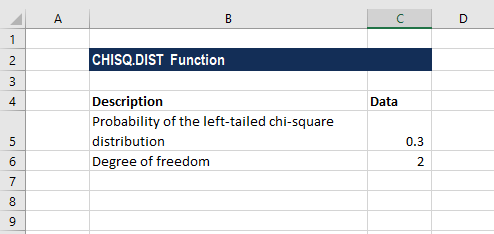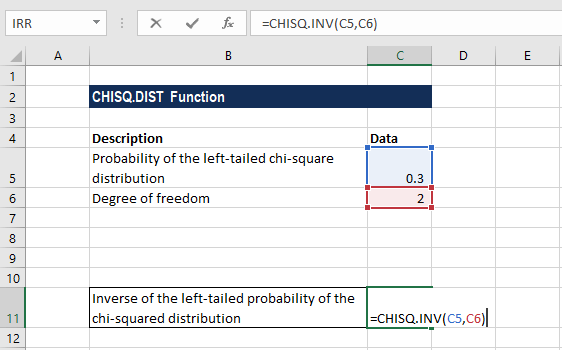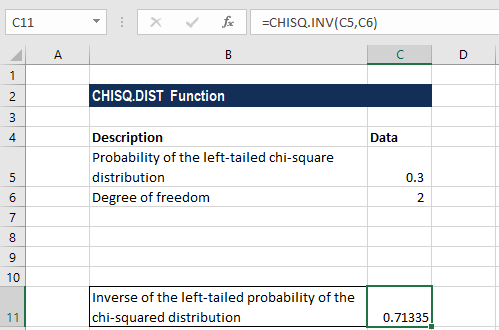# CHISQ.INV Function

Calculates the inverse of the left-tailed probability of the chi-square distribution

## What is the CHISQ.INV Function?

The CHISQ.INV Function is categorized under Excel Statistical functions. It will calculate the inverse of the left-tailed probability of the chi-square distribution.

In financial analysis, this function can be useful in finding out the variations in assumptions made. For example, consider a precision machine that produces balls for a high-quality ball bearing with a diameter of precisely 2000 μm (2 mm). Assuming that the machines typically produce balls of that size on average, but individual balls can vary slightly in either direction following a normal distribution.

Depending on production conditions, such as the raw material supplied, humidity factor, etc., production will vary so that some machines produce balls distributed around the target of 3000 μm, while others produce balls with a wider distribution. Therefore, the variance parameter of the normal distribution of the ball sizes varies from machine to machine. Using CHISQ, we can model the variance of a particular machine.

### Formula

=CHISQ.INV(probability,deg_freedom)

The CHISQ.INV function uses the following arguments:

1. Probability (required argument) – This is the probability associated with the chi-squared distribution.
2. Deg_freedom (required argument) – This is the number of degrees of freedom. It must be an integer between 1 and 1010.

### How to use the CHISQ.INV Function in Excel?

To understand the uses of the CHISQ.INV function, let us consider an example:

#### Example 1

Suppose we are given the following data:The formula for calculating the inverse of the left-tailed probability of the chi-squared distribution is shown below:We get the result below:So, the inverse of the left-tailed probability of the chi-squared distribution is 0.71335.

### Things to remember about the CHISQ.INV Function

1. If the argument deg_freedom is not an integer, it is truncated by MS Excel.
2. #VALUE! error – Occurs when any of the arguments provided is non-numeric – that is, MS Excel is unable to recognize them as numerical values.
3. #NUM! error – Occurs when:
• The probability value is less than zero or greater than or is equal to one.
• The degrees_freedom argument is less than 1 or greater than 1010.
4. The CHISQ.INV function was introduced in Excel 2010 and hence is unavailable in earlier versions.

CFI is the official provider of the FMVA Financial Modeling Certification, designed to transform anyone into a world-class financial analyst.

Thanks for reading CFI’s guide to important Excel functions! By taking the time to learn and master these functions, you’ll significantly speed up your financial analysis. To learn more, check out these additional CFI resources:

• Excel for Finance – Formulas and Functions